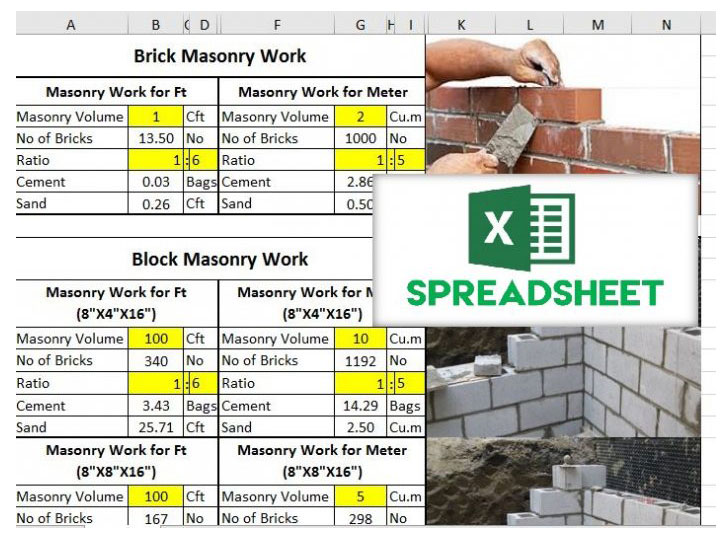When you are a civil engineer, site engineer, or similarly engaged in construction work directly, you have to know exactly how much material you need for the work or you cannot do your job. For this, a quantity calculation sheet comes very handy. So, here we are with the civil work quantities excel sheet for free download.

## Civil Work Quantity Calculation

The work of constructing a building is divided into many parts such as concreting, masonry, plastering, tiles etc. Each of this work requires a certain number of materials to construct. For example, for concrete work you need to know exactly how much cement, fine aggregates, and coarse aggregates do you need. Also, you need to know the mass of the steel you need for reinforcing.

This is what we know as the civil work quantity calculation. It is possible to do this work by hand, but it would be a huge task. To make it easier, we present a very useful excel workbook for civil work quantity. This workbook has sections to calculate the material quantity for each type of work.

But not only that, there is another sheet inside the workbook that is a converter sheet. Using this sheet, you can convert any quantity from one unit to another unit. For example, you can convert how many cubic feet of concrete you need to cubic meters.

You can do the following kinds of work using this civil work quantities excel sheet.

## Brick Masonry Work:

The following quantities of brick masonry work you can find out using this sheet.

1. Masonry Volume
2. No of Bricks
3. Ratio
4. Cement
5. Sand

## Concrete Work:

1. Concrete Work for Feet
2. Concrete Work for Meter
3. Steel in RCC per Meter
4. Steel in RCC per Foot

## Plaster Work:

1. Plaster Work per Foot
2. Plaster Work per Meter

## Tiling work:

1. Tile Work per Foot
2. Tile Work per Meter

## Unit conversion:

This sheet is great for converting all types of units from one type to another. All the units supported for conversion in this sheet are:

• Meter to feet
• Feet to Meter
• Area
• Sft to Sq.m
• Sq.m to Sft
• Feet to Centimeter
• Inch to Centimeter
• Inch to Millimeter
• Sooter to Millimeter
• Mile to Km
• Km to Mile
• Mile to Meter
• Mile to Feet
• Km to Meter
• Km to Feet
• Sq.in to Sq.mm
• Ton to Kg
• Kg to Ton
• Kg to Lbs.

## How to calculate brickwork per square meter including mortar

Assuming

• Grade of mortar = 1:6 (cement to sand)
• Class A brick (19 cm x 9 cm x 9 cm)
• Volume of brickwork = 1.0 m3
• Thickness of mortar = 10 mm

## Step 1. Calculation of bricks

No. of bricks = (volume of brick work / volume of one brick with mortar)
Volume of one brick (without mortar) = 0.19 x 0.09 x 0.09 = 0.001539 m3
Since thickness of mortar = 10 mm (0.01 m)
Volume of brick with mortar = (0.19+0.01) x (0.09+0.1) x (0.09+0.1) = 0.2×0.1×0.1 = 0.002 m3
Therefore,
No. of bricks = 1.0/ (0.002) = 500
consider the percentage of waste as 10 % or 15 %
Total no. of bricks = 500 + (10 x 500)/100=550

## Step 2. Calculation for quantity of mortar

Since we need 500 no of bricks
Volume occupied by bricks = No of bricks x volume of one brick
Volume of bricks = 500 x 0.001539 = 0.7695 m3
Volume of mortar = Volume of BRICK WORK – Volume of BRICKS
Therefore,
Volume of Mortar = 1.0 – 0.7695 = 0.2305 m^3

## Step 3. Calculation for Quantity of Cement

Cement = (dry volume of mortar x Cement ratio)/ sum of the ratios proportion)
Dry volume of Mortar = 1.33 x 0.2305 = 0.3065 m3 (33% increment due to volume shrink after water)
Cement= (0.3065 x 1) / (1+6) = 0.3065/7= 0.043795 m^3
Cement (kg) = 0.043795 x 1440 = 63.0648 kg
No. of bags = 63.0648 / 50 = 1.26 bags approximately 2 bags

## Step 4. Calculation for Quantity of Sand

Sand = (dry volume of mortar x Sand ratio)/ sum of the ratio proportion)
or
Sand = cement volume x 6 (since 1:6 grade of mortar)
Therefore,
Sand = 0.043795 = 0.26277 m3, or
Sand = 0.26277 x 1920 = 504.5184 kg /1000 = 0.5045 tons or
Sand = 0.26277 x 35.3147 = 9.279 cft or
Sand = 0.26277 x 0.354 = 0.0930 brass

## Results

So, For
Grade of mortar = 1:6 (cement to sand)
Class A brick (19 cm x 9 cm x 9 cm)
Volume of brickwork = 1.0 m3
Thickness of mortar = 10 mm
Number of Bricks = 500
Cement = 1.26 bags of 50 Kg
Sand = 0.26277 m3

Hope this is useful!